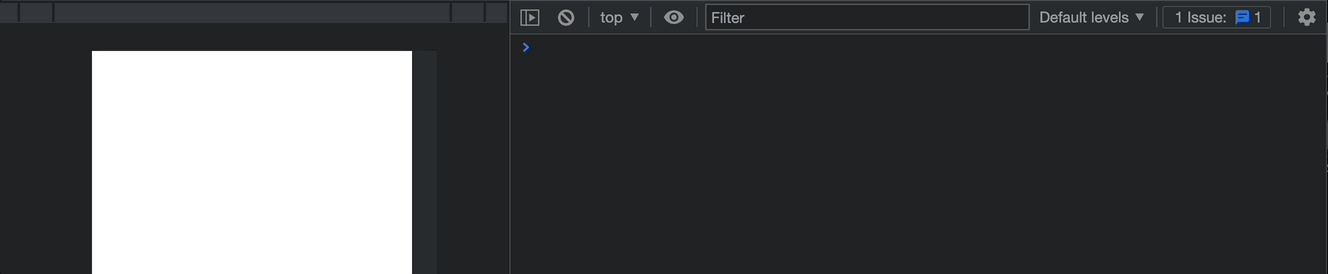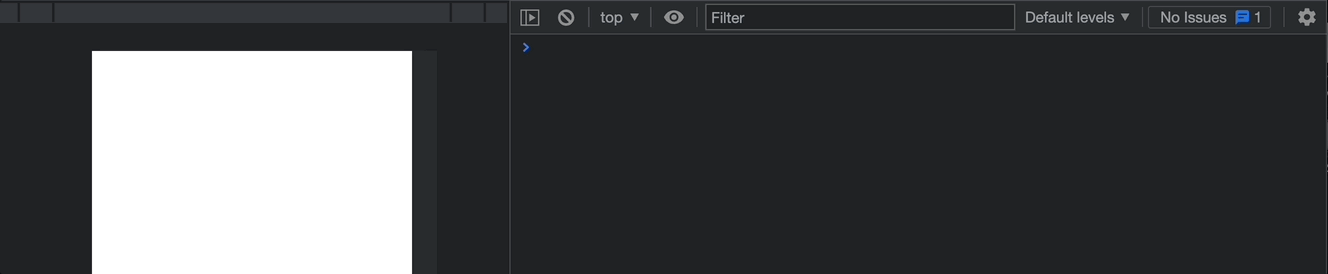# What is Memoization? Explained With JavaScriptHave you ever packed your backpack for school in the morning? You know how long it can take to pack everything you need for the day. But what if you didn't have to pack your backpack every morning? What if you could remember what you packed the day before and add what you need today? That would save you a lot of time, right?

Well, that's kind of like what memoization is in computer programming. It's a way to remember the results of a function so that the function doesn't have to be run again for the same input. This makes the function run faster and more efficiently, just like if you could remember what you packed yesterday, you could save time today.

In this article, you will understand memoization and learn how it works with JavaScript, which is similar to other languages.

## What is Memoization?

Memoization is a technique used in computer programming to optimize the performance of a function. When a function is called with a particular input, the function computes the output based on the input.

Memoization involves storing the output of a function so that when the function is called with the same input again, the stored output is returned instead of recomputing the output.

Here's an example: imagine you have a function that calculates the factorial of a number. The factorial of a number is the product of all positive integers up to that number. So the factorial of 5, for example, is 5 x 4 x 3 x 2 x 1, which equals 120.

If you call the factorial function with an input of 5, it will calculate the factorial of 5 and return 120. But what if you call the function again with an input of 5? It will have to compute the factorial of 5 again, even though it already did that the first time. This can slow down your code and use more CPU resources than necessary.

Calculating the factorial of 5 may not be an issue if it keeps re-calculating the output, but what if you perform a very expensive computation that takes time? Then performing the same calculation over and over seems unwise 🙂.

For example, the function below is used to calculate the square of a number:

``````const square = (n) => {
let result = 0;
for (let i = 1; i <= n; i++) {
for (let j = 1; j <= n; j++) {
result += 1;
}
}
return result;
};
``````

Although this may not be the best way to write the code, let’s use this because this is an expensive operation as you are looping twice — the worst-case complexity is O(n²).

When you use values like 5, the return may still be fast, but when you use large values like 3000, it lags — you would certainly not want to perform this multiple times.## How Does Memoization Work?

Memoization works by storing the output of a function for a particular input so that the function doesn't have to be run again for the same input. This is kind of like storing things in a treasure chest.

Once you've computed the output of a function for a particular input, you store that output in a treasure chest so that you can easily retrieve it later if you need it again.

Here's an example of how memoization works in JavaScript:

``````const memoizedValaues = [];

const square = (n) => {
if (memoizedValaues[n]) return memoizedValaues[n];
let result = 0;
for (let i = 1; i <= n; i++) {
for (let j = 1; j <= n; j++) {
result += 1;
}
}
memoizedValaues[n] = result;
return result;
};
``````

In the code above, you create an empty array called "memoizedValues". This array will store the results of previous calculations so that the function can avoid recalculating them in the future.

The function first checks if the calculation result for the input "n" is already stored in the "memoizedValues" array. If it is, the function returns the cached value instead of computing it again. This is called memoization.

If the result is not already stored in the "memoizedValues" array, the function computes the result by looping over two nested loops that iterate from 1 to "n". The result of each iteration is added to a variable called "result". After computing the result, the function stores it in the "memoizedValues" array at the index corresponding to the input "n". Finally, the function returns the computed result.

So the idea is that if the function is called again with the same input "n", it can skip the computation and return the cached result, which is much faster than computing it from scratch.You can decide to create a memoization function that works for any function in your program you wish to memoize:

``````const memoizeFn = (fn) => {
const cache = {};
return (...args) => {
if (cache[args]) {
return cache[args];
}
const result = fn.apply(this, args);
cache[args] = result;
return result;
};
};
``````

In this example, you define a function called `memoize` that takes a function `fn` as an argument. The `memoize` function returns a new function that wraps the original function (`factorial` in this case).

``````const factorial = (n) => {
if (n === 0 || n === 1) {
return 1;
}
return n * factorial(n - 1);
};

const memoizedFactorial = memoizeFn(factorial);
``````

The new function checks if the output for the input arguments already exists in a cache object. If it does, it returns the cached result. If it doesn't, it calls the original function and stores the result in the cache object before returning the result.

``````console.log(memoizedFactorial(5)); // 120
``````

So when you call `memoizedFactorial(5)` for the first time, it computes the factorial of 5 and stores the result (120) in the cache object. When you call `memoizedFactorial(5)` the second time, it retrieves the cached value and returns without performing any computation.

The same function can be used for the square example:

``````const square = (n) => {
let result = 0;
for (let i = 1; i <= n; i++) {
for (let j = 1; j <= n; j++) {
result += 1;
}
}
return result;
};

const memoizedSquare = memoizeFn(square);

console.log(memoizedSquare(30000)); // 900000000
``````

## Why is Memoization Useful?

Memoization is useful because it can significantly improve the performance of a function. By caching the output for a particular input, you can avoid recomputing the output every time the function is called with the same input.

In addition to improving performance, memoization can make code easier to read and understand. By separating the caching logic from the original function, you can keep the original function focused on its primary responsibility without cluttering it with caching code.

## Conclusion

In this article, you have learned what Memoization means, how it works, and how you can implement it with JavaScript. You have also learned that it can significantly improve performance and reduce the amount of CPU resources required to run functions.

Have fun coding!### Written by Joel Olawanle

Joel is a software engineer and teacher. He write's about software development, productivity, and life. He has written over 150 technical content that has helped millions of people all over the world.